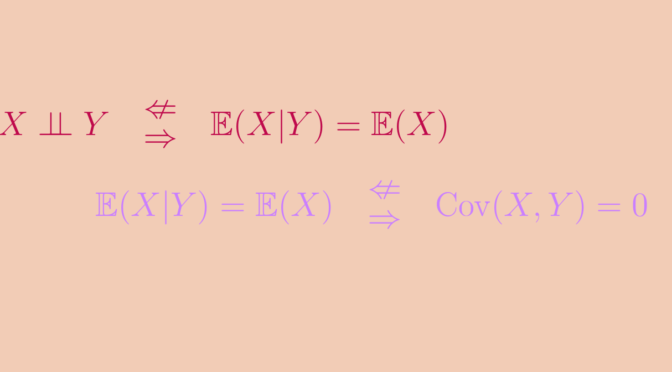# Mean independent and correlated variables

Given two real random variables $$X$$ and $$Y$$, we say that:

1. $$X$$ and $$Y$$ are independent if the events $$\{X \le x\}$$ and $$\{Y \le y\}$$ are independent for any $$x,y$$,
2. $$X$$ is mean independent from $$Y$$ if its conditional mean $$E(Y | X=x)$$ equals its (unconditional) mean $$E(Y)$$ for all $$x$$ such that the probability that $$X = x$$ is not zero,
3. $$X$$ and $$Y$$ are uncorrelated if $$\mathbb{E}(XY)=\mathbb{E}(X)\mathbb{E}(Y)$$.

Assuming the necessary integrability hypothesis, we have the implications $$\ 1 \implies 2 \implies 3$$.

The $$2^{\mbox{nd}}$$ implication follows from the law of iterated expectations: $$\mathbb{E}(XY) = \mathbb{E}\big(\mathbb{E}(XY|Y)\big) = \mathbb{E}\big(\mathbb{E}(X|Y)Y\big) = \mathbb{E}(X)\mathbb{E}(Y)$$

Yet none of the reciprocals of these two implications are true.

## Mean-independence without independence

Let $$\theta \sim \mbox{Unif}(0,2\pi)$$, and $$(X,Y)=\big(\cos(\theta),\sin(\theta)\big)$$.

Then for all $$y \in [-1,1]$$, conditionally to $$Y=y$$, $$X$$ follows a uniform distribution on $$\{-\sqrt{1-y^2},\sqrt{1-y^2}\}$$, so: $\mathbb{E}(X|Y=y)=0=\mathbb{E}(X).$ Likewise, we have $$\mathbb{E}(Y|X) = 0$$.

Yet $$X$$ and $$Y$$ are not independent. Indeed, $$\mathbb{P}(X>0.75)>0$$  and $$\mathbb{P}(Y>0.75) > 0$$, but  $$\mathbb{P}(X>0.75, Y>0.75) = 0$$ because $$X^2+Y^2 = 1$$ and $$0.75^2 + 0.75^2 > 1$$.

## Uncorrelation without mean-independence

A simple counterexample is $$(X,Y)$$ uniformly distributed on the vertices of a regular polygon centered on the origin, not symmetric with respect to either axis.

For example, let $$(X, Y)$$ have uniform distribution with values in $\big\{(1,3), (-3,1), (-1,-3), (3,-1)\big\}.$

Then $$\mathbb{E}(XY) = 0$$ and $$\mathbb{E}(X)=\mathbb{E}(Y)=0$$, so $$X$$ and $$Y$$ are uncorrelated.

Yet $$\mathbb{E}(X|Y=1) = -3$$, $$\mathbb{E}(X|Y=3)=1$$ so we don’t have $$\mathbb{E}(X|Y) = \mathbb{E}(X)$$. Likewise, we don’t have $$\mathbb{E}(Y|X) = \mathbb{E}(Y)$$.